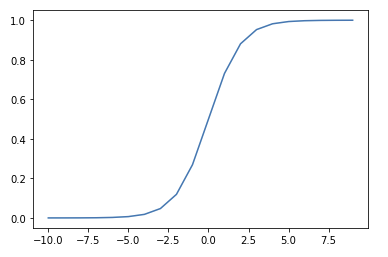## Sigmoid Activation FunctionThe Sigmoid function squeezes numbers into a probability-like range between 0 and 1.1 Used in Binary Classification model architectures to compute loss on discrete labels, that is, labels that are either 1 or 0 (hotdog or not hotdog). The equation is:

$S(x) = \frac{1}{1 + e^{-x}}$

Intuitively, when x is infinity ($e^{-\infty}=0$), the Sigmoid becomes $\frac{1}{1}$ and when x is -infinity ($e^{\infty} = \infty$) the Sigmoid becomes $\frac{1}{inf}$. That means the model is incentivised to output values as high as possible in a positive case, and low for the negative case.

Fox and Guestrin (2016)

It is named Sigmoid because of its S-like Function Shape. Its name combines the lowercase sigma character and the suffix -oid, which means similar to.

It can be described and plotted in Python, as follows:

1. Technically, there are many Sigmoid functions, each that return different ranges of numbers. This function's correct name is the Logistic Function. An alternative function that outputs values between -1 and 1 is called the Hyperbolic Tangent. However, in Machine Learning, sigmoid always refers to the Logistic Function.

#### References

Emily Fox and Carlo Guestrin. Machine Learning: Classification. 2016. URL: https://www.coursera.org/learn/ml-classification?specialization=machine-learning.